# Base Class Pointer and Derived Class Object in C++

## Base Class Pointer and Derived Class Object in C++ with Examples:

In this article, I am going to discuss Base Class Pointer and Derived Class Object in C++ with Examples. Please read our previous article where we discussed Generalization and Specialization in C++ with Example.

##### Base Class Pointer and Derived Class Object in C++:

A base class pointer can point to a derived class object in C++, but we can only access base class members using the base class pointer. Now let us understand the base class pointer and drive class object in C++ with examples. For a better understanding, please have a look at the below code. So here we have created a class called Base. In this class, we have three functions fun1(), fun2() and fun3(). For a better understanding, please have a look at the below image.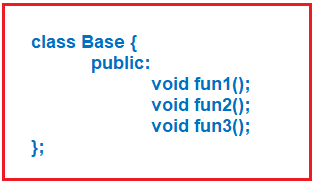Next, we have created the Derived class that is publically inheriting from the Base class. This class has also some member functions that are fun4() and fun5(). For a better understanding, please have a look at the below image.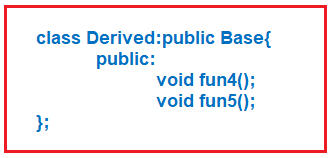Now let us write the main function as follows. Here we have the main function. Inside the main function, we have created an object b of class Base. Now on this b object, what are the functions that we can call? fun1(), fun2() and fun3() are the functions we can call. This is because all these functions are present inside the Base class. For a better understanding, please have a look at the below image.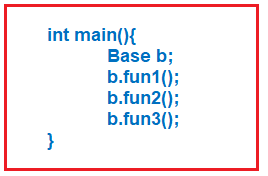Now instead of the Base class, let us create an object Derived class as follows. Here we have created an object d of the Derived class. Now, what are the functions that can we call through d? As it is inheriting from the Base class so fun1, fun2, and fun3 are already present in the Base class as well as fun4 and fun5 are present in the Derived class. So total 5 functions we can call using the derived class object d. For a better understanding, please have a look at the below image.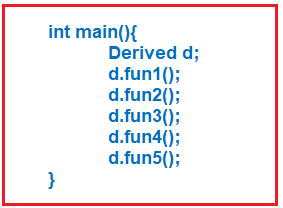This is possible because the Derived class is inherited from the Base class in C++. This is called inheritance and we already know this. Now, we are going to make changes here as follows. Here we have written the main function again with some changes. Here we have taken a Base class pointer p. We can take a pointer of any type. A pointer is a variable that can store the address. Next, we assigned p to the object of the Derived class. For a better understanding, please have a look at the below image.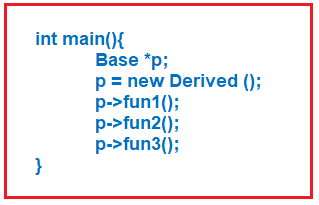##### Is it possible that a base class pointer pointing to a Derived class object in C++?

Yes, it is possible.

Next, we have called functions fun1, fun2, and fun3 with the help of pointer p. The question is which functions will be called? Pointer is of one class and the object is of another class. Whose functions will be called? Base class functions will be called.

##### Can we call fun4 and fun5?

No, we cannot call these functions.

The point that we learn here is that you can have a base class pointer and a derived class object attached to it and you can call only those functions which are present in the base class. You cannot call the functions which are defined in the derived class. But the object is a derived class object. So, you can call only those functions which are present in the base class because the pointer reference or pointer is the base class.

##### Example to Understand Base Class Pointer and Derived Class Object in C++
```#include <iostream>
using namespace std;

class Base
{
public:
void fun1()
{
cout << "fun1 of Base Class" << endl;
}
void fun2()
{
cout << "fun2 of Base Class" << endl;
}
void fun3()
{
cout << "fun3 of Base Class" << endl;
}
};

class Derived:public Base
{
public:
void fun4()
{
cout << "fun4 of Derived Class" << endl;
}
void fun5()
{
cout << "fun5 of Derived Class" << endl;
}
};

int main()
{
Base *p;
p = new Derived ();
p->fun1 ();
p->fun2 ();
p->fun3 ();

//The following statements will throw error
//p->fun4 (); //error: ‘class Base’ has no member named ‘fun4’;
//p->fun5 (); //error: ‘class Base’ has no member named ‘fun5’;
}
```
###### Output: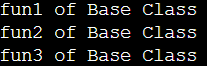##### Real-time Example to understand Base Class Pointer and Derived Class Object in C++

Let us understand Base Class Pointer and Derived Class Object in C++ with one real-time example. Suppose we have two classes Rectangle and Cuboid and Cuboid is inheriting from the Rectangle class as follows.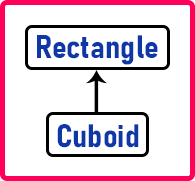Then we created the pointer of base class Rectangle which is pointing to a derived class object i.e. Cuboid as follows.
Rectangle *r;
r = new Cuboid();

Now can we have a pointer of Rectangle pointing to the object of Cuboid? Yes. The conceptual meaning if you pick out from this one is, you have a cuboid drawn here,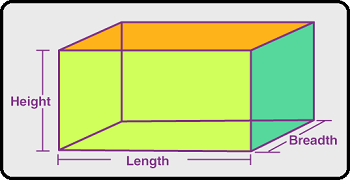Now a person says that it is a rectangle. Is he wrong? No, he is right. The person is right. The person is not defining it completely but he is saying it is rectangular. So, can an object of the cuboid have a pointer of the base class that is a rectangle? Yes, this is allowed.

And once you said it’s a Rectangle, it means you don’t know that it’s a Cuboid. Why? You don’t know that it is having height. You don’t know that you can calculate volume. That’s the meaning. Then what do you know when you are seeing a Rectangle? You know how to calculate area and you know how to calculate perimeter. So that’s it. You can go to only those functions that are present in the Rectangle class. You cannot call the functions of the Cuboid class.

Now, let us give you one more conceptual example to understand this concept better. Suppose there is a basic Car. A basic car means having the basic features of a car. Nothing is automated, no extra features, except for driving a car there are no extra things like there is no air conditioner, no media player, and no keyless entry. Nothing is there.

Then you have an advanced car. And an advanced car is inheriting from a basic car. So, can we have a pointer of a basic car, and to that pointer, can we assign an abject of an advanced car? Yes.

Then using the basic class pointer which functions you can call? You can call only the functions which are present in the basic car. It is just like you know basic car but there is an advanced car here with all the extra features in the car which you have no idea.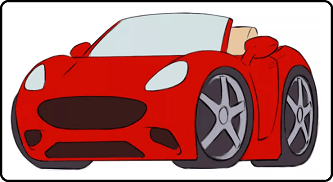This is our advanced car but I’m saying that this is a basic car. Am I right or wrong? I’m right. It’s more than a basic car. But what I said is it is a basic car. So, I’m not wrong but when I think that is a basic car, do you think I can drive it like an advanced car? I don’t know the advanced features. I know only basic features.

So, when you have a pointer to the base class, then you can call only those functions which are present inside the base class in C++. You cannot call the functions of the derived class. That’s it.

##### Can we create a Derived pointer assigned to the Base class object in C++?

Please have a look at the below code. Here, we are creating the Derived class pointer p and assigning it with the base class object.
Derived *p;
p = new Base();

Is it possible that we have a derived class pointer and I have assigned the object of the base class? No, not possible. Why? Let us see the reason.

See we have a basic car. Now if we are calling the basic car as an advanced car. Then can we get the advanced car features in the basic car? The answer is No. So, many of the features of our advanced car are not available in the basic car. If I think that it is an advanced car, I cannot use the features. Suppose if I tried keyless entry then it is not there, if I try to open moon roof then it’s not there, if I try to start AC then it’s not there.

That means the methods of an advanced car are not present in the basic car. So, you cannot call a basic car as an advanced car but you can call an advanced car as a basic car. Now, let us see some more programming examples to understand this concept in a better manner.

##### Example1: We cannot access derived class functions using the base class pointer in C++.
```#include <iostream>
using namespace std;

class Base
{
public:
void fun1()
{
cout << "fun1 of Base Class" << endl;
}
};

class Derived:public Base
{
public:void fun2()
{
cout << "fun2 of Derived Class" << endl;
}
};

int main()
{
Base *p;
p = new Derived();
p->fun1();
//The following statement will give compilation error
p->fun2(); //error: ‘class Base’ has no member named ‘fun2’; did you mean ‘fun1’?
return 0;
}
```
###### Output: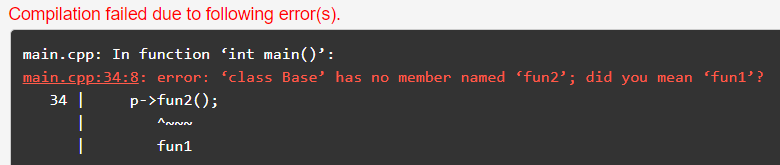Here we got an error because we are accessing derived class function i.e. ‘fun2 ()’ through the base class pointer.

##### Example2: Rectangle and Cuboid Example
```#include <iostream>
using namespace std;

class Rectangle
{
public:
void Area()
{
cout << "Area Function of Rectangle" << endl;
}
void Perimeter()
{
cout << "Perimeter Function of Rectangle" << endl;
}
};

class Cuboid:public Rectangle
{
public:
void Volume()
{
cout << "Volume Function pf Cuboid" << endl;
}
};

int main()
{
Rectangle *r;
r = new Cuboid();
r->Area();
r->Perimeter();
//The following function call will give compilation error
r->Volume(); //error: ‘class Rectangle’ has no member named ‘Volume’
return 0;
}
```
###### Output: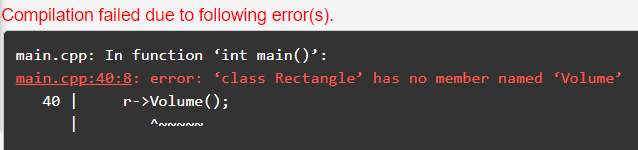The same error we are getting here. We cannot call derived class functions through the base class pointer. Now if we create a Cuboid (derived class) pointer and point it to Rectangle (base class) object as shown in the below example.

##### Example3: Derived Class Pointer and Base Class Object in C++
```#include <iostream>
using namespace std;

class Rectangle
{
public:
void Area()
{
cout << "Area Function of Rectangle" << endl;
}
void Perimeter()
{
cout << "Perimeter Function of Rectangle" << endl;
}
};

class Cuboid:public Rectangle
{
public:
void Volume()
{
cout << "Volume Function pf Cuboid" << endl;
}
};

int main()
{
Rectangle r;
Cuboid *c = &r;
c->Area();
c->Perimeter();
c->Volume();
return 0;
}
```
###### Output: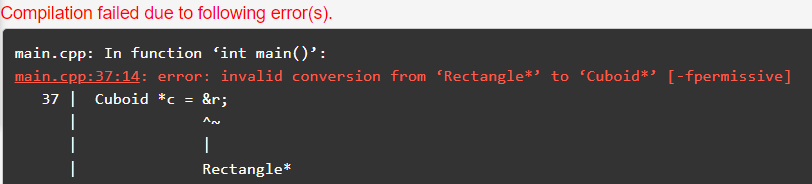Here we are getting an error “invalid conversion from ‘Rectangle *’ to ‘Cuboid*’”, it is because we can say a Cuboid is Rectangle but we cannot say a Rectangle is a Cuboid.

##### Example4: Basic Car and Advanced Car Example
```#include<iostream>
using namespace std;

class BasicCar
{
public:
void Start()
{
cout << "Car Started" << endl;
}
};

{
public:
void PlayMusic()
{
cout << "Playing Music" << endl;
}
};

int main()
{
BasicCar *ptr = &a;
ptr->Start();
//The following statement will throw compilation error
ptr->PlayMusic(); //error: ‘class BasicCar’ has no member named ‘PlayMusic’
return 0;
}
```
###### Output: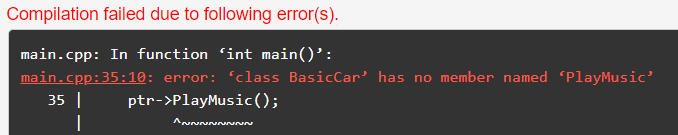Here we got an error for calling AdvanceCar (Derived class) PlayMusic function through BasicCar (Base class) pointer. Now if we create an AdvanceCar pointer and assigned it to the BasicCar object as shown in the below example.

```#include<iostream>
using namespace std;

class BasicCar
{
public:
void Start()
{
cout << "Car Started" << endl;
}
};

{
public:
void PlayMusic()
{
cout << "Playing Music" << endl;
}
};

int main()
{
BasicCar b;
ptr->Start();
ptr->PlayMusic();
return 0;
}
```
###### Output: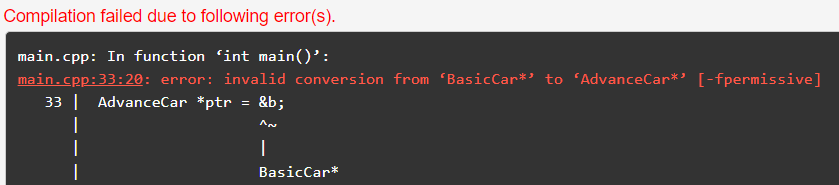So again, like in the previous example, here we got an error that “Invalid conversion from BasicCar to AdvanceCar” because Basic Car is not an advance car but an advance car is a basic car as well as the advance car.

1.2.